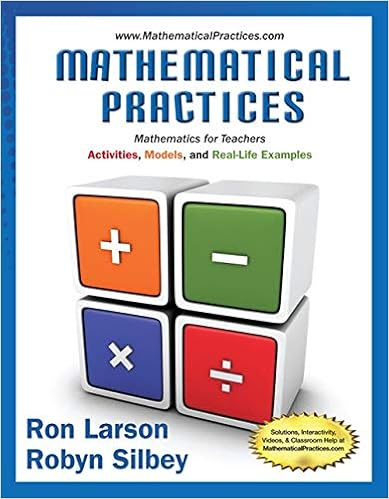MAP103 PracticeFinal F14 - Stony Brook University...

• Test Prep
• 3
• 100% (3) 3 out of 3 people found this document helpful

This preview shows page 1 - 2 out of 3 pages.

We have textbook solutions for you!
The document you are viewing contains questions related to this textbook.The document you are viewing contains questions related to this textbook.
Chapter 9 / Exercise 26
Mathematical Practices, Mathematics for Teachers: Activities, Models, and Real-Life Examples
LarsonExpert Verified
Stony Brook University Proficiency Algebra Mathematics Department MAT 103, Fall 2014 Julia Viro Practice Final Exam Final exam will consists of two parts: Part I and Part II. Part I will cover the material we studied between Midterm 2 and Final. Part II will be commulative. Part I 1. For each of the following expressions state if it is a polynomial or not. For polynomials, indicate their degrees. a) 6 x 5 - x 4 + 2 x 3 - 4 x + 5 b) 6 x 5 - x 4 + 2 x 3 - 4 x + 5 c) 6 x 5 - x 4 + 2 x 3 - 4 x + x d) 6 x 5 - x 4 + 2 x 3 - 4 x + 5 3 2 e) 6 x - 5 - x - 4 + 2 x - 3 - 4 x - 1 + 5 2. Simplify the following expressions: a) 3 75 2 - 5 27 b) 3 64 · 250 c) (1 - 7)(1 + 7) d) 3(2 3 + 6) e) ( - 3 2 x ) 2 f) ( - 3 8 x 2 ) 3 3. Express in simplest radical form a) 1 1 + 2 b) 9 3 3 3 c) 2 + 3 2 - 3 4. Solve the following quadratic equations: a) x 2 - 16 = 0 b) x 2 + 16 = 0 c) x 2 - 8 = 0 d) - 3 x 2 + x = 0
We have textbook solutions for you!
The document you are viewing contains questions related to this textbook.The document you are viewing contains questions related to this textbook.
Chapter 9 / Exercise 26
Mathematical Practices, Mathematics for Teachers: Activities, Models, and Real-Life Examples
LarsonExpert Verified
•••